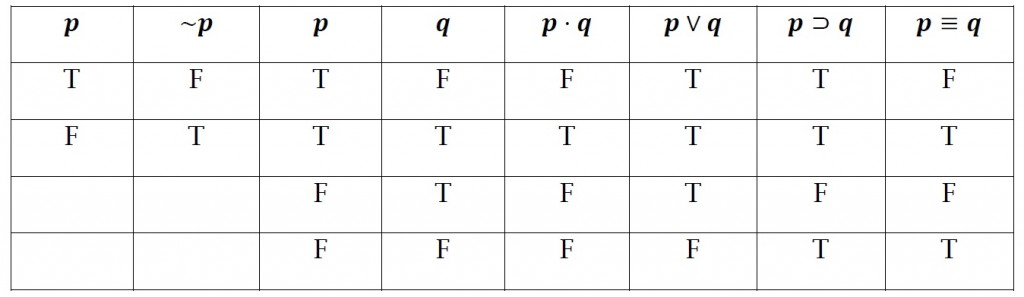## Formal logic

#### Truth-functional axioms and operators

It is assumed:

• that every proposition is either true or false
• that no proposition is both true and false

Truth and falsity are called the truth values of propositions.

If the truth value of a proposition can be determined given the truth values of the arguments and the operator which forms the proposition, then the proposition is called the truth function of the operator’s argument and the operator is called a truth functional operator.

The truth functionality of operators is set out below for any two propositions p and q:

• the truth function, “not p”, is false when p is true, and true when p is false
• the truth function, “p and q”, is true when p and q are both true, and as false in all other cases
• the truth function, “p or q”, is false when p and q are both false, and true in all other cases
• the truth function, “if p then q”, is false when p is true and q is false, and true in all other cases
• the truth function, “p is equivalent to q” is true when p and q are both true or when both are false, and false when p and q have different truth values

and also in the table below, where,

• true is “T”;
• false is “F”;

all possible combinations of truth values of the operators’ arguments are listed to the left of the vertical line.### Laws of thought

The laws of thought are the three fundamental laws of logic, and these are:

• the law of non-contradiction
• the law of excluded middle
• the principle of identity

Pages: 1 2 3 4 5